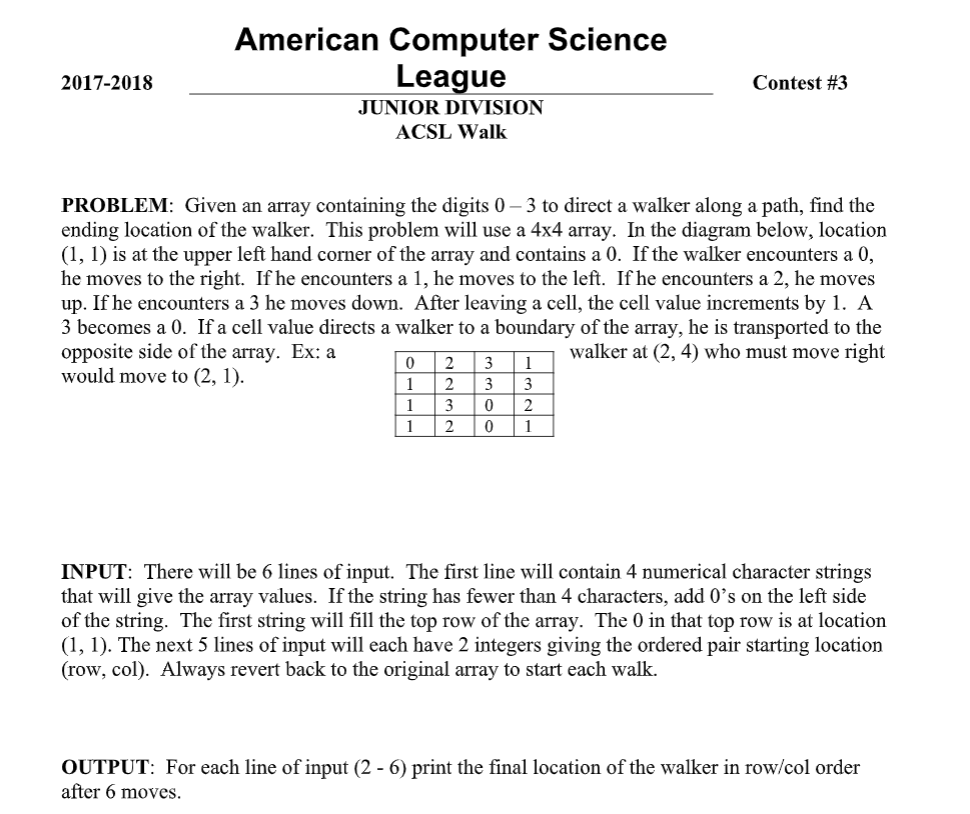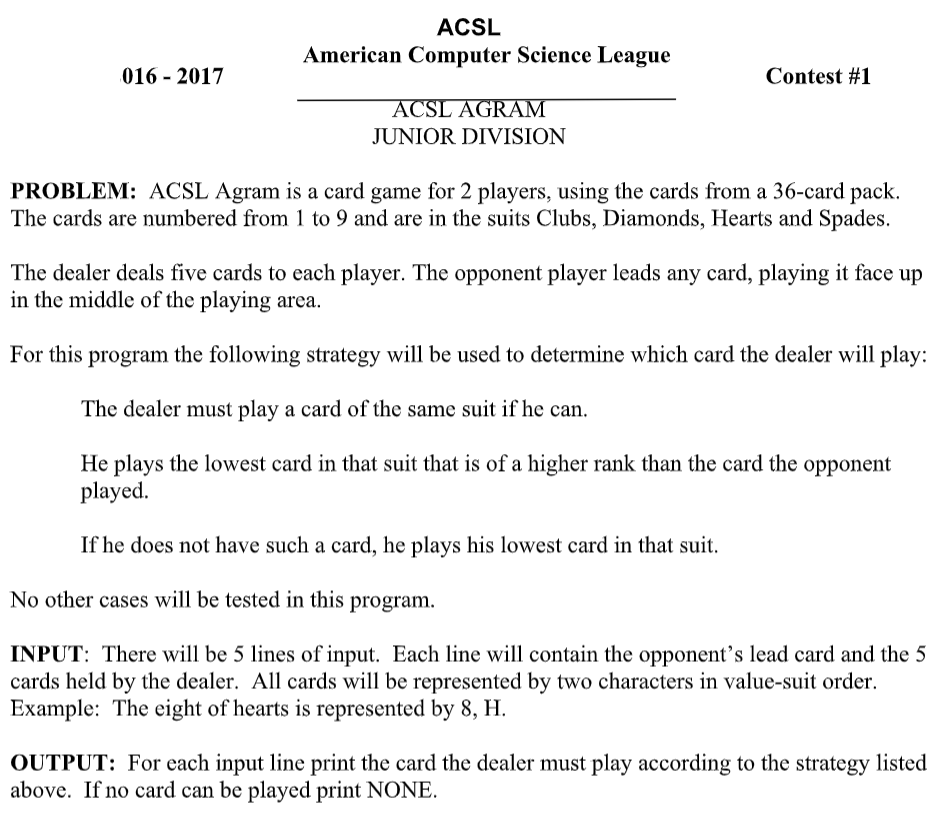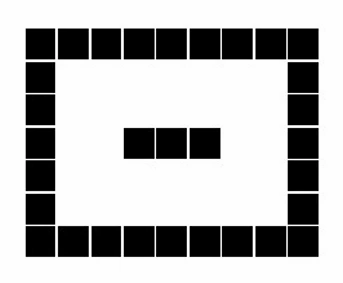## Forum Posts

Nov 09, 2020
In CS Fundamentals for ACSL
Complete the review by next week, but complete the BSTs and Heaps anytime in the next 3 weeks (we will have the local contest next week, then thanksgiving break, and then the next class). Review: Since this is for studying for the local contest, I'll post many different problems along with their answers. Do as many as you think you need to be ready. There are 5 total questions and 30 minutes to do them so if you will have 6 minutes on average to do each question. The topics that will be on the local contest are computer number systems (2 questions), recursive functions (2 questions), and What does this program do (1 question). Topics to know: How to count in binary, octal, hex Converting from binary, octal, or hex to decimal Converting from decimal to binary, octal, or hex Converting from octal or hex to binary (without going to decimal) Converting from binary to octal or hex (without going to decimal) Addition, subtraction, multiplication, and division in binary Doing calculations with mixed bases Solving recursive functions with 1 or 2 variables How to analyze code involving if statements (What does this program do will not involve loops or arrays) Computer number systems (the number in brackets indicates the base): How many 1's are in the binary representation of 16743(8)? Convert 3F6A(16) to octal Which of these has the most 1's in its binary representation? 414(8) 1B5(16) 178(10) 200(16) 600(8) How many 1's are in the solution to this expression? (743(8) – AF(16) + 110100101000(2) ) * 256(10) What is the solution to this expression in hexadecimal? (23(8) + A3(16) )/ 2(10) Which is the largest? F1(16) 375(8) 10F(16) 264(10) 11111000(2) Simplify the following and convert the answer to binary: 101(2) * 3(10) + 11(16) – 52(8) / 6(10) Answers: 9 37552 1B5 7 5B 10F 11001 Recursive functions: Find f(12) Find f(9) Find f(10,2) Find f(100) Find f(1,10) Answers: 44 11 19 0 32 55 What does this program do? Answers: 6 34 304 9 BSTs and Heaps: Make a binary search tree and a heap out of the word KEYBOARD. In the replies, tell me the depth, internal path length, external path length, how many external nodes there are, and which nodes are leaf nodes for both the BST and the heap. In case you need a refresher, overviews of all topics can be found here: http://www.categories.acsl.org/wiki/index.php?title=Main_Page0
1
35
Nov 04, 2020
In CS Fundamentals for ACSL
1. Finish this problem we started in class: Sample input: (2+3*6+1 2-5*(6+1 5+5–2)*5 3*5+(8/4*2 2+8/4*5) Sample output: 7, 9 9 1, 3 9, 11 1, 3, 5 Test input: 6 + 2 / 3 * 4 ) ( 2 – 2 + 2 + 3 * 4 / 2 8 / ( 2 + 3 – 6 + 2 7 – 5 + 8 * 6 / 2 ) + 1 9 + 6 ) / 2 – 4 + 5 Test output: 1, 3, 5 5, 7, 9, 11, 13 7, 9, 11 1, 3, 5, 7 1 2. Evaluate the next item popped in this sequence if it were a queue, and then if it were a stack: PUSH(C) POP(X) PUSH(U) PUSH(Y) POP(X) PUSH(X) PUSH(H) PUSH(G) PUSH(A) PUSH(Q) POP(X) POP(X) PUSH(N) PUSH(L) POP(X) PUSH(E) PUSH(F) POP(X) POP(X)1
4
35
Oct 27, 2020
In CS Fundamentals for ACSL
Finish the coding problem we started in class Here is the code we started in Python: Taking input: board = [] line = input().split(", ") i = 0 for i in range(0,4): board.append([]) while len(line[i])<4: line[i] = "0"+line[i] for j in line[i]: board[i].append(int(j)) Moving the walker once: copy = [row[:] for row in board] #this creates a copy of the list board coords = input().split(", ") r = int(coords)-1 c = int(coords)-1 if copy[r][c]==0: copy[r][c] = (copy[r][c]+1)%4 c = (c+1)%4 elif copy[r][c]==1: copy[r][c] = (copy[r][c]+1)%4 if c==0: c = 3 else: c-=1 elif copy[r][c]==2: copy[r][c] = (copy[r][c]+1)%4 if r==0: r = 3 else: r-=1 elif copy[r][c]==3: copy[r][c] = (copy[r][c]+1)%4 r = (r+1)%4 Here is the code we started in Java: Taking input: Scanner in = new Scanner(System.in); int[][] board = new int; String[] line = in.nextLine().split(", "); for(int i=0; i<4; i++){ while(line[i].length()<4){ line[i] = "0" + line[i]; } for(int j=0; j<4; j++){ board[i][j] = line[i].charAt(j)-'0'; } } Moving the walker once: int[][] copy = new int[]; for(int j=0; j<4; j++){ copy[j] = board[j].clone(); #this creates a copy of the array board } String[] coords = in.nextLine().split(", "); int r = Integer.parseInt(coords)-1; int c = Integer.parseInt(coords)-1; for(int j=0; j<6; j++){ if(copy[r][c]==0){ copy[r][c] = (copy[r][c]+1)%4; c = (c+1)%4; } else if(copy[r][c]==1){ copy[r][c] = (copy[r][c]+1)%4; if(c==0) c = 3; else c--; } else if(copy[r][c]==2){ copy[r][c] = (copy[r][c]+1)%4; if(r==0) r = 3; else r--; } else if(copy[r][c]==3){ copy[r][c] = (copy[r][c]+1)%4; r = (r+1)%4; }0
2
38
Oct 19, 2020
In CS Fundamentals for ACSL
1. Finish the coding problem we started in class Sample input: 5, D, 2, D, 6, H, 9, D, 9, S, 6, H 4, C, 1, C, 6, C, 7, H, 5, S, 4, D 3, D, 4, H, 5, C, 6, S, 2, D, 1, D 9, S, 8, H, 7, C, 7, D, 9, H, 3, H 1, C, 1, D, 9, H, 8, S, 9, S, 7, C Sample output: 9, D 6, C 1, D NONE 7, C Test input: 4, S, 2, S, 5, S, 6, S, 8, S, 9, S 7, H, 3, S, 3, H, 3, D, 3, C, 2, H 9, D, 3, C, 5, H, 1, S, 7, D, 9, S 6, C, 1, S, 2, H, 7, S, 8, D, 9, H 1, D, 2, S, 3, D, 4, S, 2, H, 2, C Test output: 5, S 2, H 7, D NONE 3, D Copy+paste your finished code as a reply to this post. 2. What is the value of f(6,3)?0
8
42
Oct 13, 2020
In CS Fundamentals for ACSL
Write a program that will take in a positive integer n, and print out the nth term of the Recaman sequence. The program must use recursion to calculate its answer. Here is a video explaining what the Recaman sequence is: https://www.youtube.com/watch?v=FGC5TdIiT9U You don't have to watch it but it helps understand what it's about. The way to calculate the nth term (represented by a(n)) is: This says that the 0th term is 0, and all other terms are the previous term plus or minus the term number. You subtract the term number if it will result in a positive number, and if the resulting number has not already been used in the sequence. Otherwise, add the term number instead. Copy+paste your program in the comments below. If you have any questions, feel free to email me. Here are some terms of the sequence, starting with the 0th term. 0, 1, 3, 6, 2, 7, 13, 20, 12, 21, 11, 22, 10, 23, 9, 24, 8, 25, 43, 62, 42, 63, 41, 18, 42, 17, 43, 16, 44, 15, 45, 14, 46, 79, 113, 78, 114, 77, 39, 78, 38, 79, 37, 80, 36, 81, 35, 82, 34, 83, 33, 84, 32, 85, 31, 86, 30, 87, 29, 88, 28, 89, 27, 90, 26, 91, 157, 224, 156, 225, 1550
3
44
Oct 05, 2020
In CS Fundamentals for ACSL
Write pseudocode that will print out an image like this. It should have an outline of black squares with an island of black squares in the middle. The input will be 2 numbers, giving the length and width of the rectangle you will need to print (both numbers are guaranteed to be 5 or greater). Your program will be able to output 3 different things, a black square, a white square, and a line break. Use these 3 "characters" to print out the entire image. The white "fringe" inside the rectangle should be equally wide in all parts of the image. In this example, the fringe is 2 squares wide at every location. It should also be as large as possible while still remaining equal, and while keeping at least some black squares in the middle island (ie: a white fringe that is only 1 square wide would not be valid, nor would a fringe that takes up the entire rectangle, with no middle island). This also means that the island should be as small as possible, but not 0. This was a long explanation so if you have any questions feel free to email me. Good luck!0
7
61
Sep 28, 2020
In CS Fundamentals for ACSL
1. What is the value of Z after this program is run? 2. Translate this pseudocode into real code: Set z = 0 and n = 1000 While n is less than or equal to 2000 repeat this code: a = n/1000 rounded down b = (n- 1000*a)/100 rounded down c = (n- 1000*a - 100*b)/10 rounded down d = n - 1000*a - 100*b - 10*c If a equals d and b equals c and a does not equal b then add 1 to z Add 1 to n Output z Paste the entire program as your response along with what it outputs. As a bonus, you can describe the purpose of the program. If you have forgotten some of the syntax, explanations and examples can be found here: http://www.categories.acsl.org/wiki/index.php?title=What_Does_This_Program_Do%3F0
7
31
Sep 21, 2020
In CS Fundamentals for ACSL
What are the results of these binary equations? Express your answer in binary as well. 11010110 + 1101101 = 11010000 – 10110011 = 1010110 × 110 = 10011010 ÷ 111 = What is the result of this mixed equation? The numbers in square brackets indicate the base of the preceding number. Express your answer in decimal. 5. 10010 * 2 ÷ (CC + 5 - 315) = The permission form for the unofficial discord can be found here: https://docs.google.com/forms/d/e/1FAIpQLSfgXVDilkz92YQtV9Gctdrj0xFdJYikUr3YeC8xFvKreTBY8A/viewform?usp=sf_link
2
7
70
Sep 14, 2020
In CS Fundamentals for ACSL
1. Convert the number 319 (in decimal) to binary, octal, and hexadecimal 2. Convert these numbers to decimal: 100011000 (in binary), 1040 (in octal), and AF5 (in hexadecimal) BONUS: What is 10110 (in binary) multiplied by 66 (in octal)? Express your answer in decimal. If you forgot any of the concepts, you can use this website as a refresher: http://www.categories.acsl.org/wiki/index.php?title=Computer_Number_Systems.
0
6
103
Aug 09, 2020
In CS Fundamentals for ACSL
What is the value of Z after this program is run? If you have forgotten some of the syntax, explanations and examples can be found here: http://www.categories.acsl.org/wiki/index.php?title=What_Does_This_Program_Do%3F0
6
39
Jul 30, 2020
In CS Fundamentals for ACSL
What are all the paths of length 3 from E to B in this graph? BONUS: Which nodes have the most paths of length 3 between them and how many are there?0
8
47